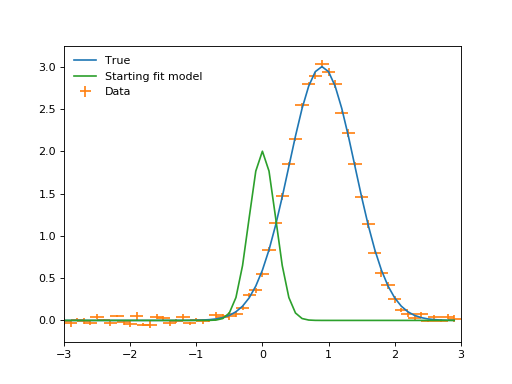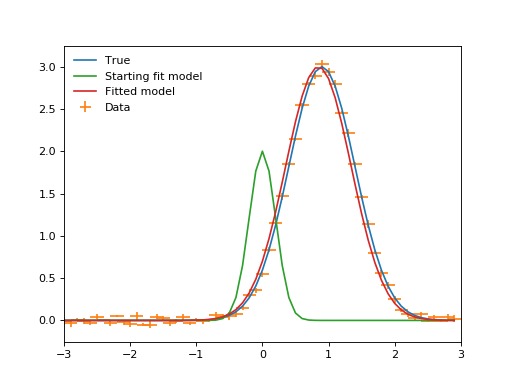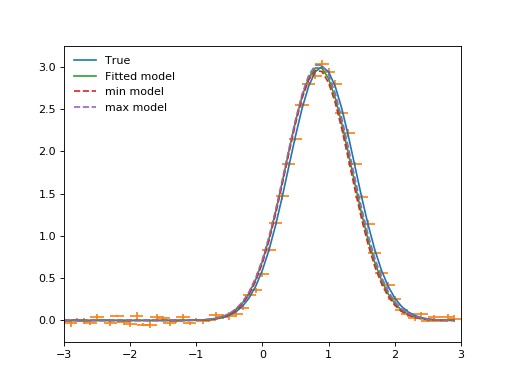# Saba: Sherpa-Astropy Bridge¶

The Saba package provides a bridge between the convenient model definition language provided in the `astropy.modeling` package and the powerful fitting capabilities of the Sherpa modeling and fitting package. In particular, Sherpa has a selection of robust optimization algorithms coupled with configurable fit statistics. Once the model fit is complete Sherpa has three different ways to estimate parameter confidence intervals, including methods that allow for coupled non-gaussian errors. Finally, Sherpa has an MCMC sampler that can be used to generate draws from the probability distribution assuming given priors.

Once Saba and Sherpa are installed, the Saba package exposes the above Sherpa functionality within the `astropy.modeling.fitting` package via a single `SherpaFitter` class which acts as a fitting backend within astropy. A plugin registry system automatically makes the `SherpaFitter` class available within the `astropy.modeling.fitting` module without requiring an explicit import.

`Saba` is the Sherpa people’s word for “bridge”.

## Installation¶

The following installation notes apply to the development version of Saba and assume use of the conda + Anaconda package system.

### Prerequisites¶

• Python 3.5+

• numpy 1.12+

• astropy 2.0 or higher (3.0 or higher recommended, as support for 2.0 will be dropped soon)

• sherpa 4.11

```conda install numpy
```

To make use of the entry points plugin registry which automatically makes the `SherpaFitter` class available within `astropy.modeling.fitting` install `astropy` version >= 1.3.

Otherwise one can just use the latest stable `astropy` via:

```conda install astropy
```

Next install Sherpa using the conda `sherpa` channel. Note that Sherpa currently needs to be installed after astropy on Mac OSX.

```conda install -c sherpa sherpa
```

Finally install `saba` using pip:

```pip install saba
```

## Getting started¶

If you are not already familiar with `astropy.modeling`, now is a good time to review the introductory documentation there along with the `astropy.modeling.fitting` module details.

To start with Saba let’s import the `SherpaFitter` class which is the interface with Sherpa’s fitting routines. `SherpaFitter` is available in one of two ways, either directly from `saba` or through `astropy.modeling.fitting` through the plugin registry system. The latter method is preferred but requires `astropy` version >= 1.3 or the latest development (master) version. Use:

```from saba import SherpaFitter
```

or

```from astropy.modeling.fitting import SherpaFitter
```

### Initialization¶

To initialize a fitter we provide string values to define the `statistic`, `optimizer` and `estmethod` (error estimation method). The available values for those can be found in the docstring of `SherpaFitter` and relate to objects within `sherpa.stats`, `sherpa.optmethods` and `sherpa.estmethods`.

```sfit = SherpaFitter(statistic='chi2', optimizer='levmar', estmethod='confidence')
```

Now that we have a fitter instance we need something to fit. So let’s import an astropy model, specifically `Gaussian1D`. A full description astropy’s model and capabilities can be found in the `astropy` Instantiating and Evaluating Models section.

```from astropy.modeling.models import Gaussian1D
```

We also need some data so let’s make some data with some added noise.

```import numpy as np
from astropy.modeling.models import Gaussian1D, Gaussian2D
import matplotlib.pyplot as plt

np.random.seed(0x1337)
true = Gaussian1D(amplitude=3, mean=0.9, stddev=0.5)
err = 0.05
step = 0.1
x = np.arange(-3, 3, step)
y = true(x) + err * np.random.uniform(-1, 1, size=len(x))

yerrs = err * np.random.uniform(0.2, 1, size=len(x))
binwidth = step * np.ones(x.shape)

fit_model = true.copy() # ofset fit model from true
fit_model.amplitude = 2
fit_model.mean = 0
fit_model.stddev = 0.2

plt.plot(x, true(x), label="True")
plt.errorbar(x, y, xerr=binwidth, yerr=yerrs, ls="", label="Data")
plt.plot(x, fit_model(x), label="Starting fit model")
plt.legend(loc=2, frameon=False)
plt.xlim((-3, 3))
plt.show()
```### Fitting¶

Now we have some data let’s fit it and hopefully we get something similar to “True” back. The `sfit` fitter object has already been initialized (as would be done for other `astropy.modeling.fitting` fitters) so we just call it with some data and an astropy model and we get the fitted model returned.

```fitted_model = sfit(fit_model, x, y, xbinsize=binwidth, err=yerrs)
plt.plot(x, true(x), label="True")
plt.errorbar(x, y, xerr=binwidth, yerr=yerrs, ls="", label="Data")
plt.plot(x, fit_model(x), label="Starting fit model")
plt.plot(x, fitted_model(x), label="Fitted model")
plt.legend(loc=2, frameon=False)
plt.xlim((-3, 3))
```

Once again plotting the data.Now we have a fit we can look at the outputs by doing:

```print(sfit.fit_info)
```
```datasets       = None
itermethodname = none
methodname     = levmar
statname       = chi2
succeeded      = True
parnames       = ('wrap_.amplitude', 'wrap_.mean', 'wrap_.stddev')
parvals        = (3.0646789274093185, 0.77853851419777986, 0.50721937454701504)
statval        = 82.7366242121
istatval       = 553.030876852
dstatval       = 470.29425264
numpoints      = 30
dof            = 27
qval           = 1.44381192266e-07
rstat          = 3.06431941526
message        = successful termination
nfev           = 84
```

Note that the `fit_info` attribute is custom to the `SherpaFitter` class and provides a direct link to the internal fitting results from the Sherpa fit process.

### Uncertainty estimation¶

One of the main drivers for Saba is to get access the uncertainty estimation methods provided by Sherpa. This is done through the `est_errors` method which uses the Sherpa’s `est_errors` method. To get the errors make a call such as:

```param_errors = sfit.est_errors(sigma=3)  # Note that sigma can be an input
```

In return we get a tuple of (`parameter_name`, `best_fit_value`, `lower_value` , `upper_value`). For the sake of plotting them we make models for the upper and lower values, and then output the values while we’re at it.

```min_model = fitted_model.copy()
max_model = fitted_model.copy()

for pname, pval, pmin, pmax in list(zip(*param_errors)):
print(pname, pval, pmin, pmax)
getattr(min_model, pname).value = pval + pmin
getattr(max_model, pname).value = pval + pmax
```
```('amplitude', 3.0646789274093185, -0.50152026852144349, 0.56964617033348119)
('mean', 0.77853851419777986, -0.096264447380365548, 0.10293940565584792)
('stddev', 0.50721937454701504, -0.098092469817728456, 0.11585973498734969)
```## Credit¶

The development of this package was made possible by the generous support of the Google Summer of Code program in 2016 under the OpenAstronomy by Michele Costa with the support and advice of mentors Tom Aldcroft, Omar Laurino, Moritz Guenther, and Doug Burke.